# Rank VS Marks Lists of students in JEE Advanced 2013 official

00:02

The marks scored by candidates in JEE Advanced 2013 is given below. This is officially released Rank vs Marks lists by IIT, Kharagpur. Note that Exam was of total 360 marks. IJEE (Advanced)  2013 had two papers; each had three parts - Physics, Chemistry, and Mathematics. In both the papers, parts 1, 2, and 3 contained 20 questions each in Physics, Chemistry, and Mathematics, respectively. The questions were numbered consecutively in both the papers. In Paper-1, each part had three sections. Section-1 had 10 multiple choice questions each having one correct answer with maximum of 2 marks with no negative marks for incorrect answer; Section-2 had 5 multiple choice questions each having one or more correct answer(s) with maximum of 4 marks and for incorrect answer -1 marks; Section-3 had 5 integer answer type questions each having maximum of 4 marks and for incorrect answer -1 marks. In Paper-2, each part had three sections. Section-1 had 8 multiple choice questions each having one or more correct answer with maximum of 3 marks; Section-2 had 8 multiple choice questions having only one correct answer with maximum of 3 marks; Section-3 had 4 multiple choice questions each having one correct answer with maximum of 3 marks. There were negative markings for wrong answers in all the Sections.
The minimum mark scored was −70 (minus seventy) and the maximum mark was 332.The rounded off average marks secured in Physics, Chemistry, and Mathematics by the candidates were 26, 22, and 20, respectively. The following are a few observations regarding the performance of candidates in JEE (Advanced) - 2013.

The following are the salient features of the result of JEE (Advanced) – 2013.
• Only 23 candidates scored above 300 marks. 326 candidates scored above 250 marks.
• The maximum marks scored out of 120 in Physics, Chemistry, and Mathematics were 120, 118, and 113 marks.
• Six candidates have scored 120 out of 120 marks in Physics.
Aggregate total marks with Different Ranks in CML (General) in JEE Advanced 2013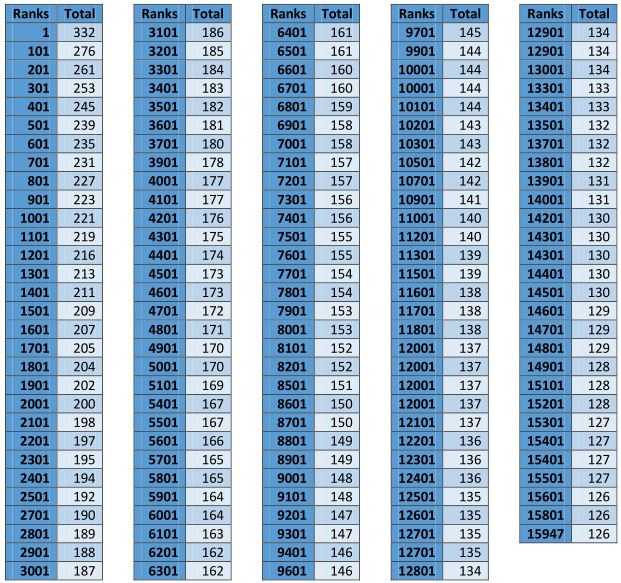Aggregate total of marks with different ranks in OBC (NCL) Category in JEE Advanced 2013Aggregate total of marks with different ranks in SC Category in JEE Advanced 2013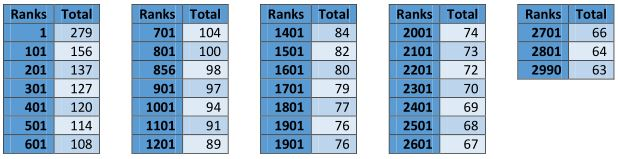Aggregate total of marks with different ranks in ST Category in JEE Advanced 2013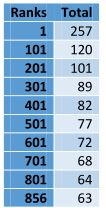Cut off Marks of all the Categories in JEE Advanced 2013

 Category Physics Chemistry Mathematics Aggregate GE 12 12 12 126 OBC(NCL) 11 11 11 113 SC,ST 6 6 6 63 PwD(All Categories) 6 6 6 63

Marks of the first and last ranked candidate in CML

 Ranks Physics Chemistry Mathematics Total 1 112 113 107 332 15947 74 40 12 126

Marks of the first and last ranked candidate in OBC (NCL) Category

 Ranks Physics Chemistry Mathematics Total 1 107 106 101 314 4188 55 45 13 113

Marks of the first and last ranked candidate in SC Category

 Ranks Physics Chemistry Mathematics Total 1 103 80 96 279 2990 27 30 6 63

Marks of the first and last ranked candidate in ST Category

 Ranks Physics Chemistry Mathematics Total 1 110 53 94 257 856 17 35 11 63

Rank VS Marks Lists of students in JEE Advanced 2014 official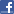Comments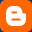0 Blogger Comments